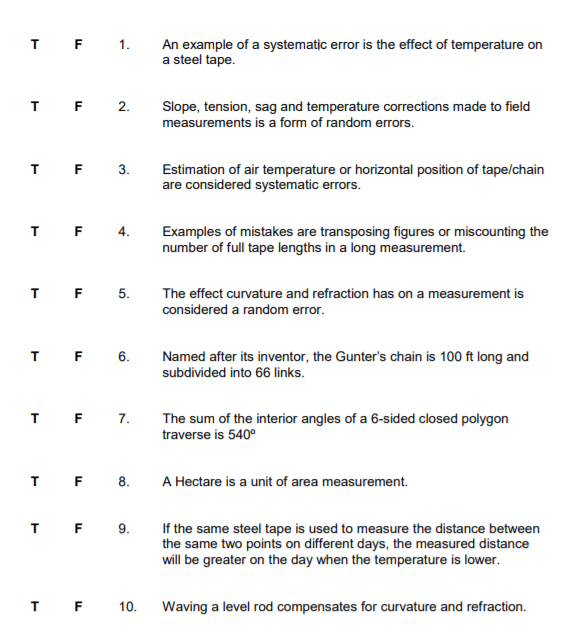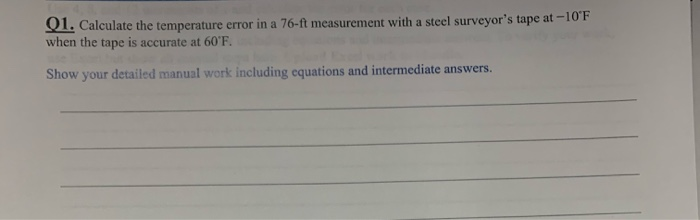# An example of a systematic error is the effect of temperature on a steel tape. 2....An example of a systematic error is the effect of temperature on a steel tape. 2. Slope, tension, sag and temperature corrections made to field measurements is a form of random errors, T F3. Estimation of air temperature or horizontal position of tape/chain are considered systematic errors. TF4.Examples of mistakes are transposing figures or miscounting the number of full tape lengths in a long measurement. The effect curvature and refraction has on a measurement is considered a random error. T F 6 Named after its inventor, the Gunter's chain is 100 ft long and subdivided into 66 links T F 7.Te sum of the interior angles of a 6-sided closed polygon traverse is 540° 8 A Hectare is a unit of area measurement. If the same steel tape is used to measure the distance between the same two points on different days, the measured distance will be greater on the day when the temperature is lower 10 Waving a level rod compensates for curvature and refraction.

solution is mentioned below with little explanation.

positive response is appreciable.

1: yes expansion of steel tapes is a source of systematic error.(happens due to temperature greater than standard)

2:slope, tension, sag and temperature correction are not random errors as these errors are cumulative in nature and can be positive or negative and is a systematic error. hence false

3: they are random in nature which can be nullified by themselves as they happen by human observations, it is not dependent on any empirical relation. hence false

4: measuring number of figure and counting chain length comes under mistake which happens due to mis- judgement and carelessness. hence true

5: curvature and refraction error are not random they both are subtractive in nature and they have set relations for finding there corrections hence it is not a random error. hence false

6: gunter chain is 66 foot long with 100 links marked of into group of 10 with brass chain.hence the given statement is false.

7: sum of angles =(n-2)*180

for six sides n=6 then overall sum =720 hence the statement is false

8:hectare is unit of measurement . 1hectare=10^4 m2 hence true

9: on the day temperature is high tape will expand more in comparison to the day when it is low. hence length of tape in higher temperature day will be greater than lower temperature day, hence lower temperature day will measure greater distance in comparison to other. hence it is true

10: no waving of rod does not compensates the error .hence the statement is false.

##### Add Answer to: An example of a systematic error is the effect of temperature on a steel tape. 2....91. Calculate the temperature error in a 76-ft measurement with a steel surveyor's tape at -10 F when the tape is accurate at 60'F. Show your detailed manual work including equations and intermediate answers.